SSAT Elementary Level Math : How to find the decimal equivalent of a fraction

Example Questions

Example Question #81 : How To Find The Decimal Equivalent Of A Fraction

What is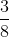equal to in decimal form?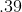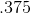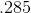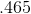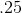Explanation:

To find the decimal equivalent of a fraction, divide the top number (numerator) by the bottom number (denominator). In this problem,divided byequalsExample Question #82 : How To Find The Decimal Equivalent Of A Fraction

What is the repeating decimal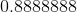.... as a fraction?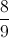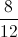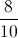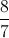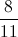Explanation:

Dividing any number by(orin multiple place values, such as the number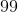or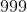) yeilds a repeating decimal: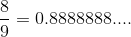Example Question #83 : How To Find The Decimal Equivalent Of A Fraction

Find the decimal equivalent to the following fraction: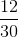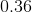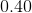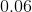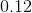Explanation:

To solve, simply reduce the fraction to a base that you can then convert easily to 100.

Once there, the numerator will be your decimal.

Thus,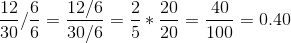Example Question #84 : How To Find The Decimal Equivalent Of A Fraction

If you ateof a pizza, what would be the decimal.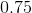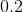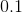Explanation:

To convert a fraction you need to put it over.

To get the denominator to, you must multiple the fraction byon the top and bottom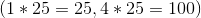.

This gives you a fraction of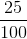and the numerator is taken and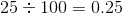.

Example Question #7 : Use Decimal Notation For Fractions With Denominators 10 Or 100: Ccss.Math.Content.4.Nf.C.6

What decimal is equivalent to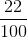?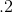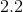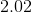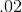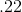Explanation:is twenty-two hundredths.is twenty-two hundredths. When we say a decimal, we say the number and add the place-value of the last digit.

Example Question #8 : Use Decimal Notation For Fractions With Denominators 10 Or 100: Ccss.Math.Content.4.Nf.C.6

What decimal is equivalent to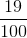?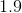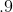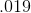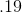Explanation:is nineteen hundredths.is nineteen hundredths. When we say a decimal, we say the number and add the place-value of the last digit.

Example Question #9 : Use Decimal Notation For Fractions With Denominators 10 Or 100: Ccss.Math.Content.4.Nf.C.6

What decimal is equivalent to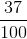?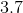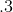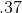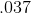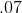Explanation:is thirty-seven hundredths.is thirty-seven hundredths. When we say a decimal, we say the number and add the place-value of the last digit.

Example Question #10 : Use Decimal Notation For Fractions With Denominators 10 Or 100: Ccss.Math.Content.4.Nf.C.6

What decimal is equivalent to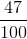?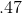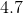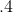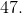Explanation:is forty-seven hundredths.is forty-seven hundredths. When we say a decimal, we say the number and add the place-value of the last digit.

Example Question #85 : How To Find The Decimal Equivalent Of A Fraction

What decimal is equivalent to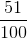?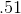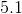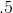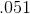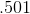Explanation:is fifty-one hundredths.is fifty-one hundredths. When we say a decimal, we say the number and add the place-value of the last digit.

Example Question #86 : How To Find The Decimal Equivalent Of A Fraction

What decimal is equivalent to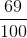?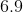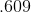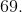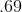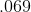is sixty-nine hundredths.is sixty-nine hundredths. When we say a decimal, we say the number and add the place-value of the last digit.